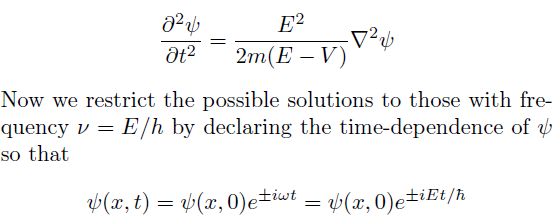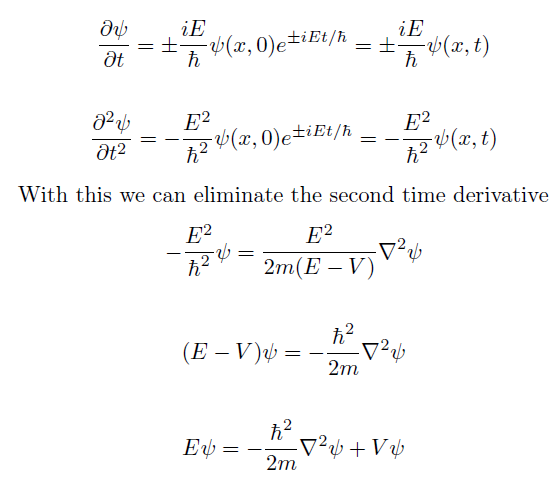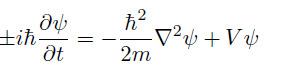Note that here Schriodinger introduces complex numbers without justi cation or comment. This was perhaps a seemingly harmless assumption because electromagnetic theory taught physicists that waves are more conveniently written in complex notation. The fi rst two time derivatives of Ψ areThis is known as the time-independent Schriodinger equation. To get the time-dependent Schriodinger equation we just substitute the rst time-derivative back inThe sign ambiguity, as Schriodinger points out, is not a problem;   Ψ obeys the equation for one sign and its